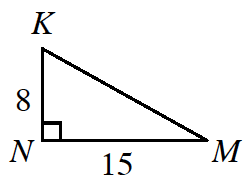### Home > GC > Chapter 2 > Lesson 2.3.1 > Problem2-101

2-101.Draw the triangle below on graph paper. Then draw a square on $\overline{KM}$ and use it to find the length of $\overline{KM}$.

Look at problem 2-96 for help with this problem.

$23^{^{2_{ }}}$$-4\left(\frac{1}{2}\right)\left(15\right)\left(8\right)$

$529-240=289.$ This is the area of the quadrilateral inside the triangles.

$\sqrt{280}=17$